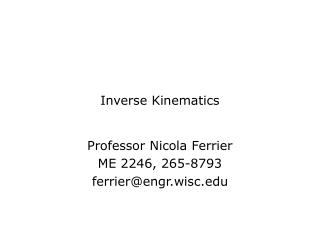Download PresentationInverse Kinematics

Inverse Kinematics - PowerPoint PPT Presentation

Inverse Kinematics. Professor Nicola Ferrier ME 2246, 265-8793 ferrier@engr.wisc.edu. Forward Kinematics. position equation: Where the transformation for each link is built from our DH parameters:. End-effector w.r.t. {e}. Forward Kinematics. Forward Kinematic Transformation:I am the owner, or an agent authorized to act on behalf of the owner, of the copyrighted work described.
Download PresentationInverse Kinematics

Download Policy: Content on the Website is provided to you AS IS for your information and personal use and may not be sold / licensed / shared on other websites without getting consent from its author.While downloading, if for some reason you are not able to download a presentation, the publisher may have deleted the file from their server.

- - - - - - - - - - - - - - - - - - - - - - - - - - E N D - - - - - - - - - - - - - - - - - - - - - - - - - -
Presentation Transcript
1. Inverse Kinematics Professor Nicola Ferrier ME 2246, 265-8793 ferrier@engr.wisc.edu

2. Forward Kinematics • position equation: • Where the transformation for each link is built from our DH parameters: End-effector w.r.t. {e}

3. Forward Kinematics • Forward Kinematic Transformation: • Has an rotation and translation: Roll,pitch,yaw Angle-axis Euler Y-Z-Y

4. Forward Kinematics • Pick one rotation description: • Decompose R, i.e. solve:

5. Forward Kinematics • Pick one rotation description: Find terms that you can easily solve equations

6. Decomposition of Rotation Matrices • Roll, Pitch, and Yaw • The inverse solution for  in (-/2,/2):

7. Decomposition of Rotation Matrices • Roll, Pitch, and Yaw • The inverse solution for  in (/2,3/2):

8. Decomposition of Rotation Matrices • Euler Angles • Inverse solution for r13 0 and r23 0 (singularity when sin=0):

9. Decomposition of Rotation Matrices • Angle Axis • Inverse solution (singularity at =0,,... and there are two solutions for §):

10. Forward Kinematics • Pick one rotation description: • Decompose T :

11. Cartesian Space & Joint Space Forward kinematics Joint Space (q1,q2,…,qN) Cartesian Space (x,y,z,,,) Inverse kinematics (arm solution)

12. Inverse Relationship? • Find joint positions for a given end-effector pose • Also find joint positions, speeds and accelerations for a sequence of poses

13. Inverse Relationship? • Analytic Inverse • Robot specific • Multiple solutions • Once found, computationally simple • Geometric • Robot specific • Insight? • Iterative Computation (ME739) • Computationally expensive • General solution

14. A Heuristic for Inverse Kinematics • The solution to the inverse kinematic equations (called the arm solution) can often be found using a heuristic approach. • Does not guarantee a solution • Solution may not be unique • Some solutions may be redundant

15. IK Heuristic Algorithm • Perform forward kinematics to find the general transformation matrix • Equate the transformation matrix to the manipulator transformation matrix. • For a particular solution the manipulator transformation matrix contains “numbers” • For a general solution the manipulator transformation matrix contains “variables”

16. IK Heuristic Algorithm • Look at both matrices for: • Elements which contain only one joint variable • Pairs of elements which will produce an expression in only one joint variable when divided (look for divisions that result in the atan2 function) • Elements, or combinations of elements, that can be simplified using trigonometric identities • Having selected an element, equate it to the corresponding element in the other matrix to produce an equation. Solve this equation to find a description of one joint variable in terms of the elements of the manipulator transformation matrix.

17. IK Heuristic Algorithm • Repeat step 4 until all the elements identified in step 3 have been used. • If any of these solutions suffer from inaccuracies, undefined results, or redundant results, set them aside and look for better solutions.

18. IK Heuristic Algorithm • If there are more joint angles to be found, pre-multiply both sides of the matrix equation by the inverse of the A1 matrix to produce a new set of equivalent matrix elements.

19. IK Heuristic Algorithm • Repeat steps 3 to 7 until either solutions to all the joint variables have been found, or you have run out of A matrices to pre-multiply. • If a suitable solution cannot be found for a joint variable, choose one of those discarded in step 6, taking note of regions where problems may occur. • If a solution cannot be found for a joint variable in terms of the elements of the manipulator transform, it may be that the manipulator cannot achieve the specified position and orientation: the position is outside the manipulator’s workspace.

20. IK Heuristic Algorithm • Note: theoretical solutions may not be physically attainable because of the mechanical limits on the range of joint variables.

21. DH Example: “academic manipulator” Z0 L2 1 x1 x2 Z2 L1 1 Z1 x0 2

22. Steps 1 and 2 • The general transformation matrix • Set equal to:

23. Steps 3 and 4 • Look for elements and select terms

24. More steps…. • Pre-multiply for a new equation:

25. DH Example: two link planar arm DH table for two link arm L2 L1 x0 x1 x2 2 1 Z2 Z0 Z1

26. Forward Kinematics: planar 2-link manipulator

27. Forward Kinematics: planar 2-link manipulator Heuristic solution? Can’t select an “obvious” set of equations…

28. Trignometric Solutions • Sometimes simple (but usually not)!

29. Trig Solutions • For first angle – revisit equations

30. Trig Solutions • A trig hint: • Let • Then • So

31. Solution to 2 link planar arm • Arm solution is • where

32. Multiple Solutions?!

33. Geometric Solutions • Intuition, insight….?

34. Geometric Solutions • Intuition, insight….?

35. Geometric Solutions • Intuition, insight….? Get same solution as trig method

36. Solution to 2 link planar arm • Arm solution is • where Here we know the sign by observation